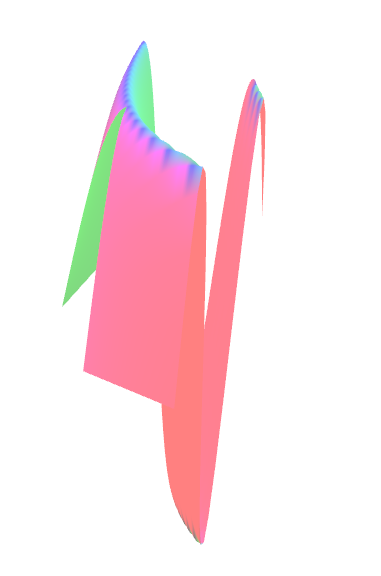0

# SurfacePlotDemo: Points set to Double.NaN cause view cutted

MarcoA 3 years ago in Defect? updated 3 years ago

I am trying to change the SurfacePlotDemo in which I want to not show a portion of the Points of the Rectangular Mesh. I tried to set the Points to Double.NaN and I can see the surface without the points but the view looks like cutted. The points that I want to exclude are excluded correctly, but the view seems to be cutted at some view angles.

If I rotate the camera the surface is shown correctlyHere is the code

public Point3D[,] CreateDataArray(Func<double, double, double> f)
{
var data = new Point3D[Rows, Columns];
for (int i = 0; i < Rows; i++)
for (int j = 0; j < Columns; j++)
{
var pt = GetPointFromIndex(i, j);
data[i, j] = new Point3D(pt.X, pt.Y, f(pt.X, pt.Y)*10);

if (i >= 0 && i < 10 && j >=0 && j < 100)
{
data[i, j] = new Point3D(double.NaN, double.NaN, double.NaN);
}

}
return data;
}

Is the only change with respect to the original example.
If I don't set any point to Double.NaN I see the surface correctly shown at any angle.

I am doing this on Windows 10 64bit, if I try on Win7 32 bit the cutting doesn't happen.

Questions:

Is setting points to NaN the correct way to not create parts of the regular mesh?

Are there any instructions to avoid this cutting?

Regards

MarcoHere is How it looks like if change view angle